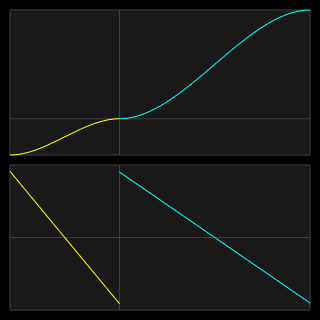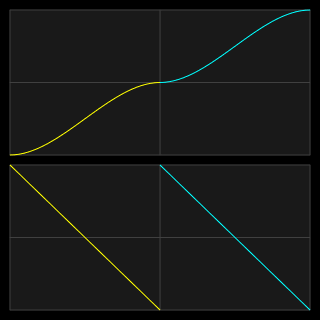## Sunday, November 11, 2018

### Interior-point constrained nonlinear optimization

Here's a boring-looking plot of something I've finally made some progress on after years of trying to learn it:This is constrained nonlinear optimization on a very tiny problem. It's a trajectory (in one spatial dimension) composed of two cubic segments (colored yellow and blue). The top plot is displacement over time, and the bottom plot is acceleration over time.

The objective is to minimize the total time of the trajectory. In these animated plots the total time is decreasing as the animation proceeds, but the plot is scaled to keep the same width so it's a bit misleading.

The cubics have fixed endpoint positions; the position at the boundary between the two cubic segments is also fixed. In this example it is closer to the left end of the curve, so the yellow cubic has a smaller displacement than the blue cubic. The velocity at the start and the end is fixed at zero, while the velocity at the boundary between the cubics is a free variable. The time durations of the two segments are the other two free variables.

The problem is constrained by limiting the maximum/minimum allowed acceleration, which is represented by the top and bottom extents of the bottom plot. Because cubics have their most extreme acceleration at their endpoints the constraints are applied there. There are eight potential acceleration constraints, because each cubic has two endpoints, and each endpoint has a lower bound and an upper bound on its acceleration.

If the midpoint between the segments is equidistant from the endpoints (as in the animated image below), then the optimum trajectory is to accelerate at maximum for the duration of the yellow segment, and then decelerate at maximum for an equal duration in the blue segment. This is a trajectory made of two parabolas, due to the constant accelerations:Because the first plot has an offset midpoint, only the shorter-duration segment can be at maximum acceleration; the blue segment needs to have a varying deceleration so it can hit the target position and velocity simultaneously. Note that if we were to break up the trajectory into more segments we could get shorter overall travel time. Here's another animation of the problem being solved, with the midpoint displacement shifted the opposite direction:Solving constrained problems like this can be done in a couple of broad ways, both of which involve taking a guess at a solution and iteratively making it better via a series of steps. In all of the examples shown my initial guess is to have the midpoint velocity be zero and the segment durations be long enough that the accelerations are within limits.

Active-set methods try to keep track of which constraints are active and move in a way that improves the objective score without violating the active constraints. As the solution is iteratively improved, the set of active constraints may change. You could think of it as sliding downward in the objective direction along the boundary of the constrained area, bumping along from surface to surface of the different constraints.

Interior-point methods, in contrast, keep all of the constraints active all the time, and instead adjust their strengths until the solution gets very close to the constraint boundary. They are kind of like landing a rocket on the surface; the solutions start out in the middle and settle down toward the constraint surface. The advantage of interior-point methods is that the structure of the problem stays the same as the solution is improved.

The animated solutions here are interior-point movement. There is a “slack” variable that controls how far from the constraint surface we're aiming to be (roughly). In the animations shown I'm controlling it by hand. I do a few iterations at a given level and when the solution looks like it's converging I reduce the slack by a factor of ten.

Both of these methods make use of first and second derivatives of the constraints and objective to come up with a likely direction to move. It involves building and solving a system of linear equations; the size corresponds to the number of variables and constraints in the problem. (It's called the Karush-Kuhn-Tucker system; there are lots of names in this area.) This gives a movement direction and expected ideal distance, which then needs to be trimmed down (backtracking) to avoid violating constraints or making things worse in any other ways.

References: